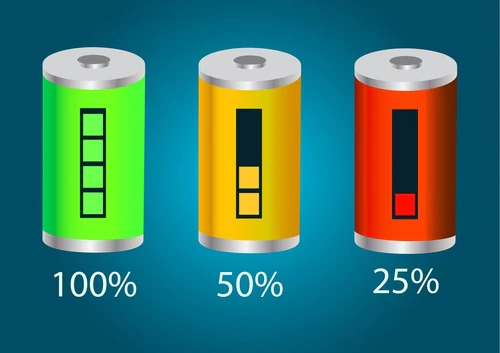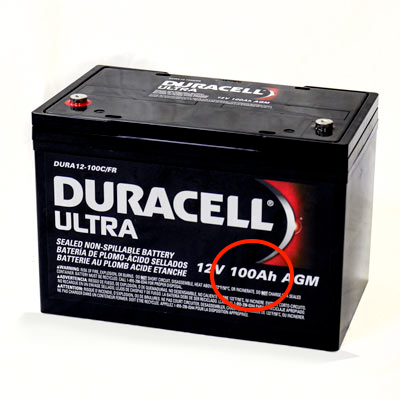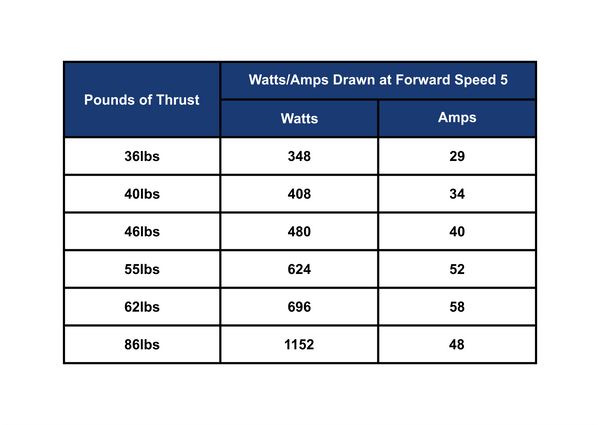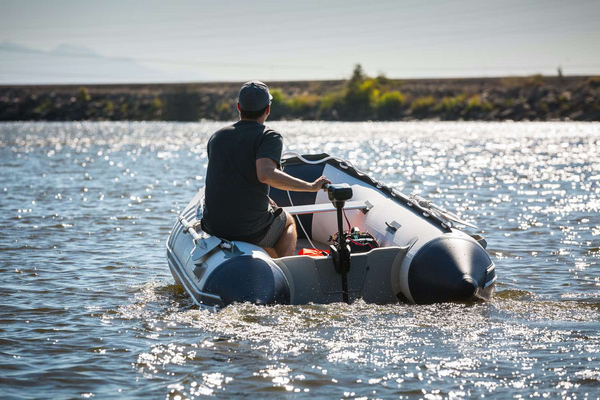## HOW LONG DOES A TROLLING MOTOR BATTERY LAST?

Two of the most common questions we receive are “How long will this motor run for?” and “How to calculate how long a battery will last?”. These are common questions because they are very important in understanding how long to stay out on the water before heading back. They are fairly easy to answer if you have all the necessary information, which can be provided by our Smart Battery Box. To determine a battery last and motor runtime, you will need to know the following information: The amperage draw of the motor and the Amp Hour rating on your battery.## AMP HOUR RATING

Amp Hour (Ah) is a common rating placed on Deep Cycle or Marine batteries. This rating measures how long a battery can maintain a consistent amperage output. More easily put, how much charge the battery can hold and how long it can power an electronic device. The larger the rating, the longer it can power a device or trolling motor.

### QUICK NOTES:

• If a battery doesn’t have an Ah rating, you can calculate the Ah rating from the Reserve Capacity (RC). You simply divide the RC by 2.4 to get an estimated amp hour rating.
• For Example: 1600 RC / 2.4 = 66.66Ah
• Cold Cranking Amps (CCA) cannot be used to estimate how long a battery will last. It measures the amount of current a given battery can deliver for 30 seconds at zero (0) degrees Fahrenheit without dropping below a specified cutoff voltage.## MOTOR AMPERAGE DRAW

Amperage draw describes the amount of electricity the motor pulls from the battery to power itself. A motor pulls different amounts of amps depending on the speed it is operating at and if there is any resistance applied to the motor. This information should be provided by the manufacturer and should be readily available on the product listing or on a specification sheet for the motor. However, only the max amperage draw will typically be listed - this is the amount current the motor pulls from the battery at top speed.

To determine how long a battery will last or how long your motor will run for, we must divide the amp hour rating on the battery against amps drawn by the motor. For example, if we have a deep cycle battery that is rated for 50Ah and we are pairing it with a motor that draws 52 Amps at max speed, we would use the following equation:

50 amp hour / 52 amps drawn = .96 hours of runtime

If the manufacturer only provides Watts drawn by the motor, we can convert this to amps by dividing against the voltage being used. You have to be careful if you are using a 12V, 24V or 36V system as this can affect your calculations. For example, if we have a 50Ah battery and a motor that draws 624 watts at full speed and uses a 12V battery, we would use the following equation:

624 watts draw / 12 volts = 52 amps drawn at top speed
50 Ah / 52 amps drawn = .96 hours of runtime

If we are using a 24V system that draws 1,152 watts with two 50Ah batteries in series (100Ah total), we would use the following equation:

1,152 watts draw / 24 volts = 48 amps drawn at top speed
100 Ah / 48 amps drawn = 2.08 hours of runtime

It should be noted that these are only estimates and they do not factor in weight, wind or water resistance or other external factors. While only estimates, these can be very helpful in deciding on the right battery or motor for your application. Below is a chart showing the max amp draw Newport Vessels trolling motors for your reference.#### QUICK NOTES:

• We can estimate amp draw based off the pounds of thrust a trolling motor is rated for by rounding down to the nearest ten.
• For example: 46lbs of thrust roughly draws 40 amps. However, this is only true for 12V powered trolling motors.
• Using a motor on lower speeds will increase battery life.
• A better estimate of this runtime can be achieved by dividing the max amp draw in half and using that answer in the equation.
• Using a motor in strong currents, winds or heavy loads will shorten the battery life.
• Just as rowing a boat up stream is harder than downstream, when a motor encounters resistance, it will pull more current from the battery to overcome the additional resistance.

#### SUMMARY

Calculating battery life is easy with two pieces of information: the battery amp hour rating, and the amount of amps a motor uses.

• The amp hour rating on a battery measures the amount of charge a battery can hold, the larger the number, the longer it can power a motor.

Motors draw different amounts of current from the battery depending on the motor size and speed it is turning. With this information, just divide the amp hour rating on your battery by the amp draw of your motor. This will give you an estimated battery life for your trolling motor.

See our selection of LiFePo4 Lithium Trolling Motor Batteries here.